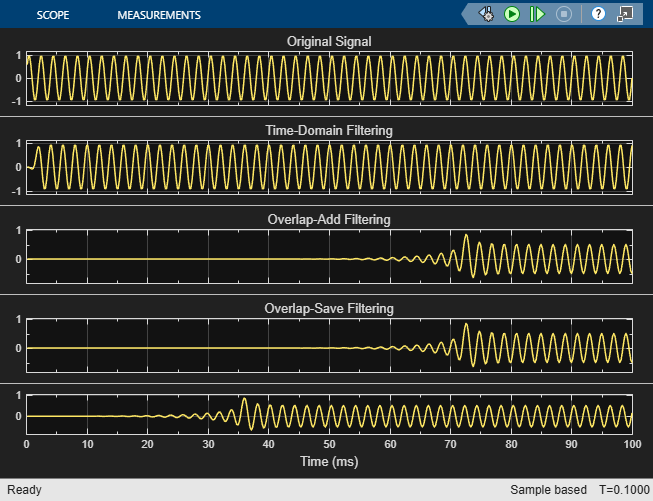This example shows how to filter a sinusoid with the Overlap-Add and Overlap-Save FFT methods using the Frequency-Domain FIR filter block.

The overlap-add algorithm  filters the input signal in the frequency domain. The input is divided into non-overlapping blocks which are linearly convolved with the FIR filter coefficients. The linear convolution of each block is computed by multiplying the discrete Fourier transforms (DFTs) of the block and the filter coefficients, and computing the inverse DFT of the product. For filter length `M` and FFT size `N`, the last `M-1` samples of the linear convolution are added to the first `M-1` samples of the next input sequence. The first `N-M+1` samples of each summation result are output in sequence.

### Overlap-Save

The overlap-save algorithm  also filters the input signal in the frequency domain. The input is divided into overlapping blocks which are circularly convolved with the FIR filter coefficients. The circular convolution of each block is computed by multiplying the DFTs of the block and the filter coefficients, and computing the inverse DFT of the product. For filter length `M` and FFT size `N`, the first `M-1` points of the circular convolution are invalid and discarded. The output consists of the remaining `N-M+1` points, which are equivalent to the true convolution.

### Latency

Overlap-save and overlap-add introduce a processing latency of N-M+1 samples. You can reduce this latency by partitioning the numerator into shorter segments, applying overlap-add or overlap-save over the partitions, and then combining the results to obtain the filtered output . The latency is reduced to the partition length, at the expense of additional computation compared to traditional overlap-save/overlap-add (though still numerically more efficient than time-domain filtering for long filters).

The model shows the results of time-domain and frequency-domain filtering of a 500 Hz sine wave. When you simulate the model, the original signal and the filtered signal are plotted both in time and in frequency domains.### Results

The first plot on the scope shows the original input signal. The second plot shows the filtered signal using conventional time-domain filtering where minimum latency is observed. The third and fourth plots show the signal filtered using the overlap-add and overlap-save frequency domain filtering methods. Both methods have the same processing latency of 213 samples. The fifth plot shows the signal filtered using the overlap-save method. The filtered signal shows a latency of 30 samples since the partition length of the overlap-save method is now 30. This partition value is less than the original partition length of 213 samples.

The Spectrum Analyzer block shows the orignal signal and the filtered signal in the frequency domain.Overlap-Add Algorithm: Proakis and Manolakis, Digital Signal Processing, 3rd ed, Prentice-Hall, Englewood Cliffs, NJ, 1996, pp. 430 - 433.

 Overlap-Save Algorithm: Oppenheim and Schafer, Discrete-Time Signal Processing, Prentice-Hall, Englewood Cliffs, NJ, 1989, pp. 558 - 560.

 T. G. Stockham Jr., "High-speed convolution and correlation", Proc. 1966 Spring Joint Computer Conf., AFIPS, Vol 28, 1966, pp. 229-233.